VI. Notes (played) on the vibrating string

## Linear Methods of Applied Mathematics Evans M. Harrell II and James V. Herod*

version of 7 May 2000

If you wish to print a nicely formatted version of this chapter, you may download the rtf file, which will be interpreted and opened by Microsoft Word or the pdf file, which will be interpreted and opened by Adobe Acrobat Reader.

## VI. Notes (played) on a vibrating string

In this chapter we separate variables in order to solve the wave equation. Many of the calculations are available in the format of a Mathematica notebook or Maple worksheet.

(Some remarks for the instructor).

There are three basic models in the subject of partial differential equations, which describe very different physics and have very different mathematical properties. They are simple in form, yet realistic for many problems in scince and engineering. Moreover, they teach us what to expect when we encounter more complicated equations used to model complicated laboratory situations. They are:

utt = c2 uxx;                                         (WE)

the heat equation, also known as the diffusion equation,

ut = k uxx;                                         (HE)

and the potential equation, also known as Poisson's equation,

uxx + uyy =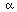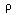(x,y),                                         (PE)

whereis a given function corresponding in electrostatics to a charge distribution, and the other quantities c, k , andare physical constants. Often there is no charge in the region where you want to measure a potential , and (PE) reduces to Laplace's equation

uxx + uyy = 0.                                         (LE)

One thing you are likely to notice if you take several advanced courses in science or engineering is that these same equations arise in many different contexts. The function u may have a different interpretation in each incarnation, but the mathematics remains the same. For example, the function u in (PE) or (LE) might be an electric potential, a magnetic potential, the height of a taut membrane, or the potential of the velocity field in a fluid.

The reason these equations arise again and again is that they are the most symmetric second order partial differential equations that can be written down. Fundamental physical principles usually do not involve derivatives of higher than second order. If a physical model is isotropic, has a superposition principle, and is based on fundamental physical principles, it is very likely to lead to an equation of one of these forms.

The mathematics and physics of the three types of equations differ in important ways, and lead to a classification of the equations as hyperbolic (WE), parabolic (HE), and elliptic (PE/LE). Other second-order equations of a given type will share the qualitative features of our three models.

We shall begin our study of the method of separation of variables with the one-dimensional wave equation, which controls the vibrations of a taut string (see derivation) and some other phenomena. The wave equation (WE) written can be written with the aid of a wave operator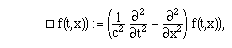so that (WE) is the equation for the kernel of this operator. This operator is also known as the d'Alembertian. The wave equation is a linear, homogeneous equation, so the superposition principle holds for its solutions. If we can find some solutions u1, ..., uk, then any linear combination of these solutions is again a solution. We also classify it as "second-order," since derivatives up to second order occur, but no higher-order derivatives. This will be the case for the great majority of PDE's in this class.

We shall solve the wave equation in two entirely different ways. The first method is known as separation of variables. Let's set c = 1 for convenience and test out the wave operator by calculating its action on some representative functions: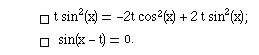Somewhat surprisingly,, the second seemingly arbitrary function is a solution of (WE)!  As we shall see, there are lots of solutions, and several strategies for finding them. With the method of separation of variables, we try to find solutions of a special form, u = T(t) X(x), called product solutions . This is a special assumption of form - what is referred to as an ansatz, to give some definiteness to our search for solutions. Some other physical circumstances, called the boundary and initial conditions, will be quite important, and will constrain us in choosing T and X; a partial differential equation coupled with some boundary conditions will be called a boundary-value problem. For the vibrating string, the most common boundary conditions are the zero Dirichlet conditions:

u(t,0) = 0, u(t,L) = 0                     (DBC)

This means that the string is fastened at two ends, x=0 and x=L. Actually, if we assigned some other fixed values to u besides 0 at the end points, we could just redefine the dependent variable:

If u(t,0) = a, u(t,L) = b,

then define v := u(t,x) - a - (b-a) x/L.

The function v will still solve the wave equation, vtt - vxx, but it will have the simpler boundary conditions (DBC).

When we act on a product T(t)X(x) with the wave operator and divide the result by T(t)X(x), we get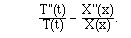(More details in these calculations are given in the accompanying Mathematica notebook or Maple worksheet.) If u solves the wave equation, this expression must be zero. (We could quibble about what happens when T or X = 0, but that would either mean that u is the uninteresting "trivial" solution or else the problem occurs only at isolated values of x and t.) Thus

```           T''/T  = X''/X.
```

Since the left side of this is independent of x and the right side is independent of t, both sides must in fact be a constant. We don't know its value yet, so let us call it -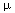. It will turn out to be negative.

-X''(x) =X(x)                               (6.1)

This sort of equation is similar to a matrix-vector equation in linear algebra and is called an eigenvalue equation. The function X and the numberare both unknown, and are called and eigenfunction and eigenvalue. We do not allow the possibility X(x) = 0 for all x. While it is always a solution for all u, it is not useful. By definition, an eigenfunction must be a nonzero solution of an equation like (6.1). Along with (6.1) we have the boundary conditions

X(0) = X(L) = 0                               (6.2)

and this will restrict the's for which (6.1) has nonzero solutions. Eq. (6.1) is an elementary ordinary differential equation with the familiar solution (also obtainable with software),

X(x) := D1 cos(1/2 x) + D2 sin(1/2 x).

Because of the boundary condition at x=0, we see that D1 = 0. We are left with only one constant, which we can scale to 1, remembering that we may want to multiply our solution by an arbitrary constant later. Because of the BC at x=0, we now know that up to a constant multiple, X(x) = sin(1/2 x). The other boundary condition in (6.2) tells us what the allowable choices forare, namely1/2 = n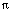/L, n = 1,2,.... Taking L=1 for convenience, the eigenfunctions and eigenvalues are:

Xn(x) := sin(nx)n := (n)2

Now let's look at the other part of the PDE which we "separated off":

T''(t) = -T(t)                               (6.3)

As we saw, there are many possible values ofwhich are consistent with the boundary condition for the function X, and the same possibilities occur in equation (6.3) (multiplyby c2 here if c is not set to 1). Therefore

Tn(t) = An cos(nt) + Bn sin(nt).

We cannot go further with the T function without being given the initial condition, that is, information about what happens at t=0.

We already have something of importance in science and engineering, however: the normal modes . Many physical systems, like the vibrating string, preferentially undergo simple motions with pure frequencies. Such a possible motion is exactly what we find by positing a product solution, imposing any boundary conditions, and solving the resulting eigenvalue problem.

Definition VI.1. A normal mode  of a boundary value problem is a solution of the form

T(t) X(x),

where X(x) incorporates all boundary conditions.

Here the spatial variable x might be more than one-dimensional, as for the problem of the vibrating drum, which will be encountered later. I have generalized the notion of a normal mode so that any time dependence is allowed, although most commonly it will be sinusoidal, T(t) = sin(t +). When it is sinusoidal, the angular frequency omega is usually a simple function of the eigenvalue.

The general solution we come up with is a linear combination of the particular solutions we get by separating the equation. It is not yet obvious that this is a completely general solution, but it is.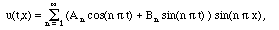(6.4)

The coefficients A and B have to be determined from the initial conditions, that is, by the condition of the string at t=0.

Suppose that

u(0,x) = f(x)

and

ut(0,x) = g(x)                               (IC)

are given, and that 0 < x < 1. Plugging in to formula (6.4) shows that: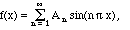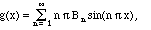In other words, A[n] are the Fourier sine coefficients for f(x), and the more complicated expression

(n) Bn

gives the Fourier sine coefficients of g(x). Notice that the stuff in the sine is nx/L and not
2 nx/L (with L=1). In other words, there are more sines in this series than in the usual Fourier series, but there are no cosines. It is precisely as if we imagine the string to be defined on the interval [-1,1], twice as long as the actual interval, but on the nonphysical part from x=-1 to x=0 we pretend that the shape of the string is defined to give us an odd function: f(-x) = -f(x). This would give us only sine functions, as we have seen before. Earlier we called those coefficients Bn, but here they happen to be called An. The thing to remember is that since they go along with sine functions (of x) the formula for them contains sines. We would thus write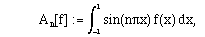but we can simplify this a bit by using the symmetry: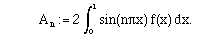What about the other coefficients? Well, this time it is the whole expression (n) Bn which is a Fourier sine coefficient for g(x), so we need to divide through by the extra factor: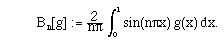Here is an example to illustrate the situation.

Model Problem VI.2. Solve the problem of the vibrating string using Fourier sine series, and initial conditions

u(x,0) = 0, ut(x,0) = 1.

Solution.

We can easily calculate the formulae for the coefficients A and B, either by hand or with software (see the Mathematica notebook or Maple worksheet):

Am = 0
and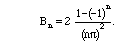If n is even, Bn = 0, else Bn = 4/(n)2

The general solution is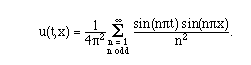Next we show some plots of the solution at various times. For computational purposes we keep terms only up to n=7. In the Mathematica notebook or Maple worksheet you can see the string vibrate by selecting the graphs and choosing "animate." By the way, infinity is larger than 7, but 7 is close enough to give us a reasonable picture of the solution: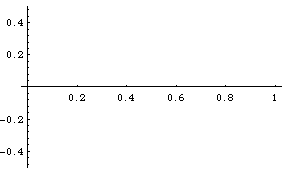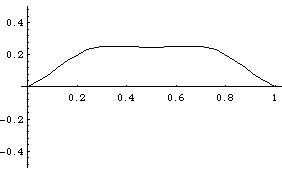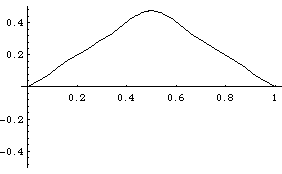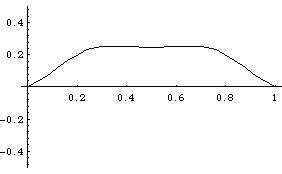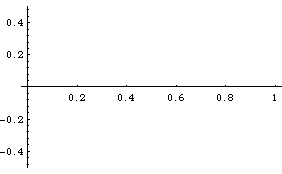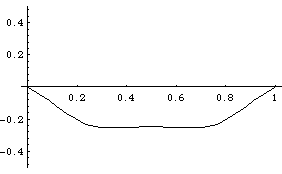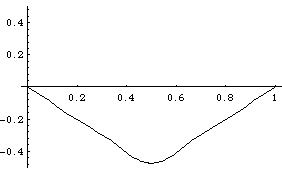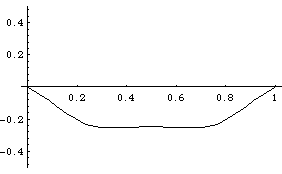The boundary conditions we have been using are not the only useful ones. Suppose that our string is attached to a pair of tracks in the vertical direction perpendicular to the x-axis using a frictionless roller. Then u(t,0) and u(t,L) are not specified, but instead we have: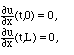(NBC)

These are called Neumann boundary conditions. Obviously, as with DBC, it would be a minor change to impose them at x=a and x=b where a does not have to be 0. At this stage, we can exactly repeat the analysis of separation of variables, until the point where we first used the boundary conditions, i.e., (6.1). With NBC, we instead evaluate the x-derivative at 0:

Model Problem VI.3. Set up the general solution of the vibrating string with Neumann BC., with the simplifications c = L = 1.

Solution.

The partial differential equation separates exactly as before, so we still know that when we assume u = X(x) T(t), we get separated equations (6.1) and (6.3). The values ofwill be different, however, because they were determined by the BC. Since as before,

X(x,) := D1 cos(1/2 x) + D2 sin(1/2 x),

we see that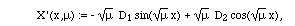and for this to be 0 when x=0, there are two possibilities, namely D1=0 or=0. (We ignored the latter possibility before since sin(0) = 0 is not a useful solution, but cos(0) = 1 can be one.)

Possibility 1.=0, which means that the output just given is not really the solution to (6.1), which has become X''(x) = 0. The solutions of this are of the form A + B x, but B has to be 0 so that X'(0) = 0. Thus with this possibility, X(x) = 1 (times any constant). Eq. (6.3) now also becomes T''(t) = 0, which leads to the product solution of (WE):

(A0 + B0 t) 1 = A0 + B0 t.

Since the boundary conditions do not apply to the t variable, we keep the general linear combination. A0 and B0 will be determined by the initial conditions.

Possibility 2.> 0. The boundary condition at x=0 now forces X(x) = cos(1/2 x) (again, up to a constant multiple), and the other boundary condition forces one of the valuesn = (n)2. The product solutions become

(An cos(nt) + Bn sin(nt)) cos(nt)

and the general solution will be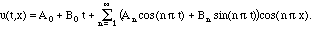(6.5)

The coefficients are determined by the initial conditions because: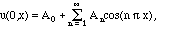and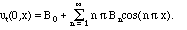The numbers An are the Fourier cosine coefficients of u[(0,x) = f(x), while B0 and nBn are the Fourier cosine coefficients of ut(0,x) = g(x). (Notice the extra factors of n.)

A realistic string or optical fiber may not be uniform, so some of the simplifying
assumptions in our derivation fo the wave equation are not valid. For example, the
constant c2 = (spring constant) / (mass density) for the spring may be different at different
positions, leading to an equation of the form

utt = (c(x))2 uxx                               (ModWE1)

or, as a thorough examination of the derivation of the wave equation reveals,we could more generally have: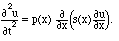(ModWE2)

for some potentially complicated positive functions p(x) and s(x). How well does the method of separation of variables do for problems like this? Rather well, actually, although we may have to encounter some new functions.

Model Problem VI.4. Suppose that the wave speed depends on position, so that
c2 = 1/(1 + x), 0 < x < 1, DBC at 0 and 1.

Find the normal modes of vibration.
Solution.

Exercises VI

1. Solve the wave equation with NBC at 0 and 1 and:

f(x) = |x-1/2|, g(x) = x2

Do you encounter any difficulties because the IC are not consistent with the BC?

2. Put DBC at x=0 and NBC at x=L. Separate variables, solve the eigenvalue problem for X(x), and write down a general solution.
3. Solve the wave equation utt = uxx with initial conditions:

f(x) = sin(x), g(x) = 0 and

a) DBC at x=0 and x=1
b) NBC at x=0 and x=1
c) DBC at x=0 and NBC at x=1

Plot the solutions and compare.

4. Solve the wave equation with IC:

f(x) = 0, g(x) = 1

and

a) DBC at x=0 and x=1
b) NBC at x=0 and x=1
c) DBC at x=0 and NBC at x=1

Plot the solutions and compare.

5. Solve the wave equation utt = uxx with initial conditions:

f(x) = sin(x), g(x) = 1 and

a) DBC at x=0 and x=1
b) NBC at x=0 and x=1
c) DBC at x=0 and NBC at x=1

Plot the solutions and compare.

6. Solve the wave equation utt = 4 uxx for 0 < x <, 0 < t <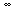, initial conditions: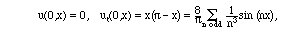and Dirichlet boundary conditions at x=0 and x=, i.e., u(t,0) = u(t,) = 0, for 0 < t.

7. Find all normal modes (product solutions) for the modified wave equation

utt + u = uxx

with Neumann boundary conditions:

ux(t,0) = 0
ux(t,) = 0

8. (Refer to the appendix for the derivation of the wave equation for this problem.) Derive a wave equation for the vibrating string if the x-axis is horizontal and we take gravity into account. The gravitational potential energy of the string is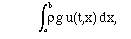where g, the gravitational constant, is approximately 980 in the cgs system.
9. Apply the method of separation of variables to the general solution of the simply supported vibrating beam problem: utt = -c2uxxxx
u(t, 0) = u(t, L) = 0, and uxx(t, 0) = uxx(t,L) = 0.
(Discussion and solution are available with or without Maple.)